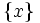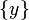# Topological indistinguishability

Jump to: navigation, search

## Definition

Topological indistinguishability is an equivalence relation on any topological space. For a topological space$X$, two (possibly equal, possibly distinct) points$x,y \in X$ are termed topologically indinstinguishable if the following equivalent conditions hold:

1. The closures of the singleton sets$\{ x \}$ and$\{ y \}$ are equal.
2. Every closed subset containing$x$ contains$y$ and every closed subset containing$y$ contains$x$.
3. Every open subset containing$x$ contains$y$ and every open subset containing$y$ contains$x$.

Two distinct points that are not topologically indistinguishable are termed topologically distinguishable.